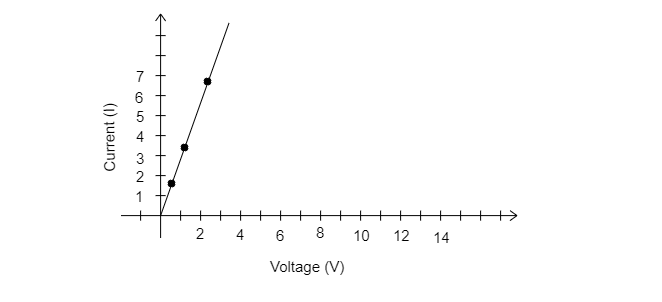I(amperes)0.51.02.03.04.0V(volts)1.63.46.710.213.2
Plot a graph between V and I and calculate the resistance of that resistor."">

# The values of current I flowing in a given resistor for the corresponding values of potential difference V across the resistor are given below ŌĆōI(amperes)0.51.02.03.04.0V(volts)1.63.46.710.213.2Plot a graph between V and I and calculate the resistance of that resistor."

Given:

The values of current I flowing in a given resistor for the corresponding values of potential difference V across the resistor are given below –

 $I(amperes)$ 0.5 1 2 3 4 $V(volts)$ 1.6 3.4 6.7 10.2 13.2

To do:

To plot a graph between V and I and calculate the resistance of that resistor.

Solution:

The graph between $V$ and $I$

On plotting the value of $V$ and $I$ on the graph, we obtain a slope as shown in the graph below:Resistance of that resistor

Resistance $R=\frac{1}{slope}$

Slope $=\frac{V_2-V_1}{I_2-I_1}$

$=\frac{1-0.5}{3.4-1.6}$

$=\frac{0.5}{1.8}$

$=0.278$

Therefore, resistance $R=\frac{1}{0.278}=3.6\ \Omega$

Updated on: 10-Oct-2022

62 Views